# Minkowski Decomposition of Convex Lattice Polygons

Angelos Mantzaflaris & Dimitris Paparas, Computational Geometry class 2007-8
contact: amantzafmath.uoa.gr  & grad0961di.uoa.gr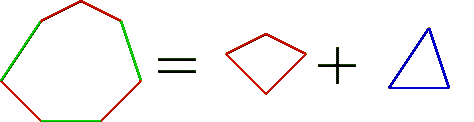Implementing python code for the computation/approximation of the decomposition of a convex lattice polygon P as P=A+B, where "+" denotes Minkowski addition.

Tools:
References:
• I.Z. Emiris, E. Tsigaridas, Minkowski decomposition of convex lattice polygons, "Algebraic geometry and geometric modeling", 2005
• S. Gao and A.G.B. Lauder, Decomposition of polytopes and polynomials, "Discrete and computational geometry", 2001
• A. Tuzikov, H.J.A.M. Heijmans, Minkowski decomposition of convex polygons into their symmetric and asymmetric parts, "Pattern Recognition Letters", 1998

Problem MINKOWSKI statement:
• Input:      A sequence of Vectors_2 defing the normal fan of a convex lattice polygon P with n vertices
(or a sequence of Points_2 defining the vertices of P)
• Output:   All  distinct sequences of Vectors_2 defining fans of summands A, where P=A+B
This problem is NP-Complete (reduction from PARTITION).

The normal fan of P is the set of vectors perpendicular to the edges u_i = p_{i+1} - p_i , i=0,...,n  and of length equal to ||u_i||, where p_i 's are the vertices of P and p_n=p_0 by convention.

The polygon P is fully defined by a vertex and the set of it's edges. For the purpose of decomposition translations are not important, so the actual size of input is determined by the bitsize of the edges (or the fan) of P rather than the bitsize of the initial polygon vertices.

## 1. Exact algorithms

### Exhaustive Search

Try all possible combinations of integer subvectors of distinct edges and output the ones that sum to zero.

A subvector of a polygon edge u_i=(u_ix, u_iy) is of the form je_i, where j is an integer 0<j<=d_i and  d_i=gcd(u_ix, u_iy). The vector e_i= u/d_i  is the primitive edge of u.

The sum of all integers j for all edges u_i equals the number of integer points on the perimeter of the polygon (also known by Pick's formula) and is related to the complexity of the problem.

### Dynamic Programming

Reduce MINKOWSKI to SUBSET SUM and use dynamic programming to solve the latter. The main steps are:

a) Define the primitive edge sequence. That is, for each primitive edge e_i=(e_x, e_y) consider the O(log d_i) vectors that are multiples of e_i with scalar factors all the powers of 2^0, 2, 2^2,... such that 2^r <= d_i/2, where d_i=gcd(u_ix, u_iy). Note that the sum of these integers does not exceed d_i (by geometric series sum). We dublicate the nessesary powers so that all these integers (resp. vectors) sum to d_i (resp. u_i).

Observe that these vectors suffice to represent any subvector of every edge of P, since every integer 1,...,d_i has a representation in the binary arithmetic system using a subset of the scalars defined before. In other words, the primitive edge sequence is a decomposition of the edges of P that generates (by addition) all subvectors of edges of P.

b) Give the set of all these vectors, that is of cardinality O(n log D) , D=max{d_i} as an input to the 2D-subset sum problem:

Input:     A set of integer vectors in the plane
Output:  One (or more) proper subset of the vectors that sums to (0,0).

Note that the input we created is polynomial in the bitsize of P, which is the input of the original MINKOWSKI problem.

c) How can we solve the 2D-subset sum problem? The problem can be easily reduced to 1D-subset sum, by considering for every (u_i,v_i) an integer u_i + Lv_i, where L is a sufficiently large integer so that it seperates the vanishing of a sum of u_i's from the vanishing of the corresponding v_i's.

The integer L should be as small as possible, since it affects the pseudopolynomial complexity factor of the subset sum problem.

The usual (1D) subset sum problem is:

Input:     A set of integers
Output:  One (or more) proper subset of the integers that sums to 0.

We initially attempted a direct algorithm for solving the 2D-subset sum problem. This used a 3D array of Vectors_2 and has been proven inefficient compared to reduction to the usual subset sum.

d) Solve the 1D-subset sum problem by dynamic programming and transform the solution back to MINKOWSKI output.

These ideas lead to a pseudopolynomial algorithm for MINKOWSKI of complexity O(n LV log D) where V=max{v_i}.

## 2. Approximation algorithms

Derive approximation algorithms based on dynamic programming. The approximation is being done to the subset sum problem using known algorithms. The approximate solution does not yield a polygon (since the corresponding vectors do not sum to zero), so it should be translated to a convex polygon that is in some sence an approximate summand of P.

The notion of an approximate solution for MINKOWSKI is to be explored.

## 3. Implementation

We have implemented the following:
• A general graphical interface using Python-CGAL-visual for the graphical input/output of polygons and decompositions. This includes an integer grid along with functions for input of integer convex polygons, functions for drawing a lattice polygon on the grid (having as input either the edge sequence or the fan of the polygon) and other graphics-related routines.
• A set of auxiliary routines that pass from edge representation to fan representation, compute the complementary summand by Minkowski substraction, compute the primitive edge sequence and several other auxiliary tasks.
• A function for the enumeration of all decompositions by exhaustive search.
• A dynamic programming algorithm for the direct solution of the 2D-subset sum problem (this has been proven inefficient compared to reduction to the usual subset sum).
• A dynamic programming algorithm for the usual subset sum problem along with routines for the reduction of MINKOWSKI input to subset sum input as well as transformation of the subset sum output to MINKOWSKI output.
• A test routine that generates the polygons used in our experiments below, executes the algorithms on them and returns results and timings.

## 4. A toy example

The following polygon has 7 distinct decompositions (computed by exhaustive search):Two-summands: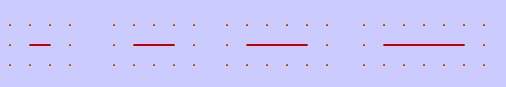One can find the complementary summand for each solution by Minkowski substraction:Three-summand:              Complementary: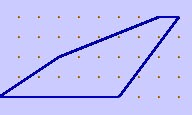Four-summands: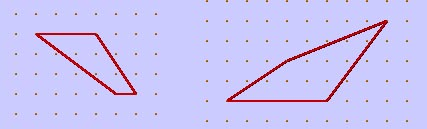ComplementariesOur Dynamic programming implementation does not return the totality of possible summands (there are exponentially many of them after all), but a subset of them, ranging from small (i.e. line summands, if they exist) to large ones. All solutions could be explored by a careful backtracking, but our intention is to discover a small amount of "interesting" decompositions rather than output an exponentially large set. In this example Dynamic programming outputs 3 decompositions: One containing line summand, one with a four-summand and one with a three-summand.

An interesting task would be to define a compact representation of all possible summands. For example instead of outputting 4 line summands,  we could denote that the two parallel edges define 4 decompositions by outputting the integer range [1,4] along with pointers to these two edges. We expect that this technique is generalizable and may lead to new algorithms for MINKOWSKI.

## 5. Experimental results

We tested our Dynamic Programming implementation on the eight possible convex canonical lattice polygons:We consider scalar multiples of these polygons, by a scalar factor k, in order to create large instances (and also hard: with a plethora of integer points on the perimeter).

For every polygon and for k ranging from 20 to 400 we list the number of decompositions discovered ("#") and the time needed ("t") in seconds on a Intel Core2Duo 2GHz machine with 1GB of main memory running Ubuntu 7.10 and Python 2.5.

 Dynamic Programming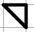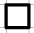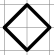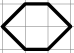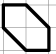k # t # t # t # t # t # t # t # t 20 5 0.011 11 0.016 11 0.060 11 0.029 11 0.073 17 0.133 17 0.091 23 0.273 40 6 0.056 13 0.072 13 0.278 13 0.136 13 0.339 20 0.688 20 0.414 27 1.290 60 8 0.171 17 0.210 17 0.864 17 0.427 17 0.991 26 1.903 26 1.300 35 3.812 80 7 0.284 15 0.358 15 1.272 15 0.671 15 1.670 23 2.889 23 1.951 31 5.803 100 8 0.406 17 0.534 17 2.232 17 1.092 17 2.747 26 5.102 26 3.300 35 10.004 120 9 0.709 19 0.910 19 3.750 19 1.950 19 4.631 29 8.478 29 5.369 39 16.914 140 9 0.936 19 1.219 19 5.087 19 2.457 19 6.228 29 11.607 29 7.720 39 22.329 160 8 1.036 17 1.364 17 6.212 17 2.887 17 7.766 26 14.274 26 9.006 35 26.784 180 10 1.620 21 2.238 21 9.398 21 4.429 21 11.725 32 20.913 32 13.515 43 40.139 200 9 1.938 19 2.427 19 11.031 19 5.289 19 13.398 29 23.769 29 15.142 39 45.751 220 11 2.765 23 3.595 23 14.869 23 7.185 23 18.192 35 32.912 35 21.688 47 65.636 240 10 2.871 21 3.808 21 16.155 21 7.813 21 19.798 32 36.619 32 23.625 43 71.561 260 9 3.080 19 4.072 19 17.159 19 8.363 19 21.089 29 38.207 29 25.282 39 76.175 280 10 3.903 21 5.159 21 22.091 21 10.669 21 26.980 32 48.893 32 32.218 43 97.786 300 11 4.968 23 6.494 23 27.730 23 13.519 23 35.191 35 61.290 35 40.402 47 135.049 320 9 6.306 19 6.879 19 26.163 19 12.704 19 32.093 29 57.906 29 38.283 39 127.395 340 11 6.344 23 10.958 23 45.373 23 17.638 23 43.604 35 90.129 35 54.920 47 175.801 360 11 7.220 23 9.363 23 39.985 23 19.497 23 58.086 35 88.505 35 58.273 47 227.275 380 13 9.661 27 12.239 27 61.804 27 25.500 27 75.913 41 126.344 41 84.147 55 380.184 400 10 8.519 21 10.589 21 45.117 21 21.997 21 55.252 32 110.953 32 68.678 43 273.083

In order to compare with exhaustive search (that returns all possible summands), we list the timings for k=1,...,7 (larger instances cannot be exhaustively searched within reasonable time):

 Exhaustive Searchk # t # t # t # t # t # t # t # t 1 1 0.000 3 0.000 3 0.000 3 0.000 3 0.001 7 0.002 9 0.002 23 0.007 2 2 0.000 8 0.001 8 0.001 8 0.001 12 0.004 34 0.014 44 0.012 236 0.121 3 3 0.001 15 0.004 15 0.004 15 0.004 27 0.132 99 0.065 135 0.065 1263 1.325 4 4 0.002 24 0.009 24 0.009 24 0.009 54 0.047 238 0.311 324 0.255 4724 7.601 5 5 0.003 35 0.018 35 0.018 35 0.018 91 0.119 479 0.869 665 0.761 13943 32.867 6 6 0.005 48 0.034 48 0.034 48 0.033 146 0.254 884 2.006 1224 2.043 34936 114.496 7 7 0.009 63 0.058 63 0.057 63 0.058 215 0.501 1487 4.398 2079 4.387 77503 352.390

Observe (from the fourth column of the above table or by an easy combinatorial argument) that a square polygon of side k has exactly k^2+2k -1 decompositions. This number is incremented by one in the table above, because the trivial decomposition is counted. In fact, exhaustive search will count each distinct decomposition twice (once for each summand), so there are ceil[ (k^2 +2k-1)/2 ] distinct decompositions of a square polygon. The "ceil" comes from the fact that when k in even there exists a "self-dual" decomposition (to two equal squares of side k/2) counted only once.

Similar formulas for the number of decompositons occur for the rest of the canonical polygons.